Request a Free Counselling Session from our Expert Mentor# NCERT Solution for Class 12 Physics Chapter 12

Welcome to the NCERT Solutions for Class 12 Physics Chapter 12 – Atoms. This chapter is an essential part of the Class 12 Physics syllabus and deals with the fundamental aspects of atomic structure. The chapter introduces the concept of the atom, the fundamental particles that make up an atom, the atomic models proposed by different scientists, and the structure of the atom. It also covers various theories and laws related to atomic structure, such as the Bohr’s model, the de Broglie hypothesis, the Heisenberg uncertainty principle, and the quantum mechanical model of the atom. This chapter is important not only from the point of view of board examinations but also from the perspective of competitive exams like JEE and NEET. In this chapter, you will learn about the fascinating world of atoms and their properties. The NCERT Solutions for Class 12 Physics Chapter 12 will provide you with a detailed explanation of all the concepts covered in the chapter and help you in understanding the subject better.

## Answers of NCERT Solution for Class 12th Physics Chapter 12 – Atoms

Page No 435:

Question 12.1:

Choose the correct alternative from the clues given at the end of the each statement:

(a) The size of the atom in Thomson’s model is ………. the atomic size in Rutherford’s model. (much greater than/no different from/much less than.)

(b) In the ground state of ………. electrons are in stable equilibrium, while in ………. electrons always experience a net force.

(Thomson’s model/ Rutherford’s model.)

(c) classical atom based on ………. is doomed to collapse.

(Thomson’s model/ Rutherford’s model.)

(d) An atom has a nearly continuous mass distribution in a ………. but has a highly non-uniform mass distribution in ……….

(Thomson’s model/ Rutherford’s model.)

(e) The positively charged part of the atom possesses most of the mass in ………. (Rutherford’s model/both the models.)

(a) The sizes of the atoms taken in Thomson’s model and Rutherford’s model have the same order of magnitude.

(b) In the ground state of Thomson’s model, the electrons are in stable equilibrium. However, in Rutherford’s model, the electrons always experience a net force.

(c) A classical atom based on Rutherford’s model is doomed to collapse.

(d) An atom has a nearly continuous mass distribution in Thomson’s model, but has a highly non-uniform mass distribution in Rutherford’s model.

(e) The positively charged part of the atom possesses most of the mass in both the models.

Question 12.2:

Suppose you are given a chance to repeat the alpha-particle scattering experiment using a thin sheet of solid hydrogen in place of the gold foil. (Hydrogen is a solid at temperatures below 14 K.) What results do you expect?

In the alpha-particle scattering experiment, if a thin sheet of solid hydrogen is used in place of a gold foil, then the scattering angle would not be large enough. This is because the mass of hydrogen (1.67 × 10−27 kg) is less than the mass of incident α−particles (6.64 × 10−27 kg). Thus, the mass of the scattering particle is more than the target nucleus (hydrogen). As a result, the α−particles would not bounce back if solid hydrogen is used in the α-particle scattering experiment.

Page No 436:

Question 12.3:

What is the shortest wavelength present in the Paschen series of spectral lines?

Rydberg’s formula is given as:

Where,

h = Planck’s constant = 6.6 × 10−34 Js

= Speed of light = 3 × 10m/s

(n1 and n2 are integers)

The shortest wavelength present in the Paschen series of the spectral lines is given for values n1 = 3 and n2 = ∞.

Question 12.4:

A difference of 2.3 eV separates two energy levels in an atom. What is the frequency of radiation emitted when the atom makes a transition from the upper level to the lower level?

Separation of two energy levels in an atom,

E = 2.3 eV

= 2.3 × 1.6 × 10−19

= 3.68 × 10−19 J

Let ν be the frequency of radiation emitted when the atom transits from the upper level to the lower level.

We have the relation for energy as:

E = hv

Where,

= Planck’s constant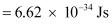Hence, the frequency of the radiation is 5.6 × 1014 Hz.

Question 12.5:

The ground state energy of hydrogen atom is −13.6 eV. What are the kinetic and potential energies of the electron in this state?

Ground state energy of hydrogen atom, E = − 13.6 eV

This is the total energy of a hydrogen atom. Kinetic energy is equal to the negative of the total energy.

Kinetic energy = − = − (− 13.6) = 13.6 eV

Potential energy is equal to the negative of two times of kinetic energy.

Potential energy = − 2 × (13.6) = − 27 .2 eV

Question 12.6:

A hydrogen atom initially in the ground level absorbs a photon, which excites it to the = 4 level. Determine the wavelength and frequency of the photon.

For ground level, n1 = 1

Let E1 be the energy of this level. It is known that E1 is related with n1 as:

The atom is excited to a higher level, n2 = 4.

Let E2 be the energy of this level.

The amount of energy absorbed by the photon is given as:

E2 − E1

For a photon of wavelengthλ, the expression of energy is written as:

Where,

h = Planck’s constant = 6.6 × 10−34 Js

c = Speed of light = 3 × 108 m/s

And, frequency of a photon is given by the relation,

Hence, the wavelength of the photon is 97 nm while the frequency is 3.1 × 1015 Hz.

Question 12.7:

(a) Using the Bohr’s model calculate the speed of the electron in a hydrogen atom in the = 1, 2, and 3 levels. (b) Calculate the orbital period in each of these levels.

(a) Let ν1 be the orbital speed of the electron in a hydrogen atom in the ground state level, n1 = 1. For charge (e) of an electron, νis given by the relation,

Where,

= 1.6 × 10−19 C

0 = Permittivity of free space = 8.85 × 10−12 N−1 C2 m−2

h = Planck’s constant = 6.62 × 10−34 Js

For level n2 = 2, we can write the relation for the corresponding orbital speed as:

And, for n3 = 3, we can write the relation for the corresponding orbital speed as:

Hence, the speed of the electron in a hydrogen atom in n = 1, n=2, and n=3 is 2.18 × 106 m/s, 1.09 × 106 m/s, 7.27 × 105 m/s respectively.

(b) Let T1 be the orbital period of the electron when it is in level n1 = 1.

Orbital period is related to orbital speed as:

Where,

r1 = Radius of the orbit

h = Planck’s constant = 6.62 × 10−34 Js

e = Charge on an electron = 1.6 × 10−19 C

= Permittivity of free space = 8.85 × 10−12 N−1 C2 m−2

m = Mass of an electron = 9.1 × 10−31 kg

For level n2 = 2, we can write the period as:

Where,

r2 = Radius of the electron in n2 = 2

And, for level n3 = 3, we can write the period as:

Where,

r3 = Radius of the electron in n3 = 3

Hence, the orbital period in each of these levels is 1.52 × 10−16 s, 1.22 × 10−15 s, and 4.12 × 10−15 s respectively.

Question 12.8:

The radius of the innermost electron orbit of a hydrogen atom is 5.3 ×10−11 m. What are the radii of the = 2 and =3 orbits?

The radius of the innermost orbit of a hydrogen atom, r1 = 5.3 × 10−11 m.

Let r2 be the radius of the orbit at n = 2. It is related to the radius of the innermost orbit as:

For n = 3, we can write the corresponding electron radius as:

Hence, the radii of an electron for n = 2 and n = 3 orbits are 2.12 × 10−10 m and 4.77 × 10−10 m respectively.

Question 12.9:

A 12.5 eV electron beam is used to bombard gaseous hydrogen at room temperature. What series of wavelengths will be emitted?

It is given that the energy of the electron beam used to bombard gaseous hydrogen at room temperature is 12.5 eV. Also, the energy of the gaseous hydrogen in its ground state at room temperature is −13.6 eV.

When gaseous hydrogen is bombarded with an electron beam, the energy of the gaseous hydrogen becomes −13.6 + 12.5 eV i.e., −1.1 eV.

Orbital energy is related to orbit level (n) as:

For n = 3,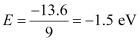This energy is approximately equal to the energy of gaseous hydrogen. It can be concluded that the electron has jumped from n = 1 to n = 3 level.

During its de-excitation, the electrons can jump from n = 3 to n = 1 directly, which forms a line of the Lyman series of the hydrogen spectrum.

We have the relation for wave number for Lyman series as:

Where,

Ry = Rydberg constant = 1.097 × 107 m−1

λ= Wavelength of radiation emitted by the transition of the electron

For n = 3, we can obtain λas:

If the electron jumps from n = 2 to n = 1, then the wavelength of the radiation is given as:

If the transition takes place from n = 3 to n = 2, then the wavelength of the radiation is given as:

This radiation corresponds to the Balmer series of the hydrogen spectrum.

Hence, in Lyman series, two wavelengths i.e., 102.5 nm and 121.5 nm are emitted. And in the Balmer series, one wavelength i.e., 656.33 nm is emitted.

Question 12.10:

In accordance with the Bohr’s model, find the quantum number that characterises the earth’s revolution around the sun in an orbit of radius 1.5 × 1011 m with orbital speed 3 × 104 m/s. (Mass of earth = 6.0 × 1024 kg.)

Radius of the orbit of the Earth around the Sun, r = 1.5 × 1011 m

Orbital speed of the Earth, ν = 3 × 104 m/s

Mass of the Earth, m = 6.0 × 1024 kg

According to Bohr’s model, angular momentum is quantized and given as:

Where,

h = Planck’s constant = 6.62 × 10−34 Js

n = Quantum number

Hence, the quanta number that characterizes the Earth’ revolution is 2.6 × 1074.

Question 12.11:

Answer the following questions, which help you understand the difference between Thomson’s model and Rutherford’s model better.

(a) Is the average angle of deflection of α-particles by a thin gold foil predicted by Thomson’s model much less, about the same, or much greater than that predicted by Rutherford’s model?

(b) Is the probability of backward scattering (i.e., scattering of α-particles at angles greater than 90°) predicted by Thomson’s model much less, about the same, or much greater than that predicted by Rutherford’s model?

(c) Keeping other factors fixed, it is found experimentally that for small thickness t, the number of α-particles scattered at moderate angles is proportional to t. What clue does this linear dependence on provide?

(d) In which model is it completely wrong to ignore multiple scattering for the calculation of average angle of scattering of α-particles by a thin foil?

The average angle of deflection of α-particles by a thin gold foil predicted by Thomson’s model is about the same size as predicted by Rutherford’s model. This is because the average angle was taken in both models.

(b) much less

The probability of scattering of α-particles at angles greater than 90° predicted by Thomson’s model is much less than that predicted by Rutherford’s model.

(c) Scattering is mainly due to single collisions. The chances of a single collision increases linearly with the number of target atoms. Since the number of target atoms increase with an increase in thickness, the collision probability depends linearly on the thickness of the target.

(d) Thomson’s model

It is wrong to ignore multiple scattering in Thomson’s model for the calculation of average angle of scattering of α−particles by a thin foil. This is because a single collision causes very little deflection in this model. Hence, the observed average scattering angle can be explained only by considering multiple scattering.

Question 12.12:

The gravitational attraction between electron and proton in a hydrogen atom is weaker than the coulomb attraction by a factor of about 10−40. An alternative way of looking at this fact is to estimate the radius of the first Bohr orbit of a hydrogen atom if the electron and proton were bound by gravitational attraction. You will find the answer interesting.

Radius of the first Bohr orbit is given by the relation,

Where,

0 = Permittivity of free space

h = Planck’s constant = 6.63 × 10−34 Js

me = Mass of an electron = 9.1 × 10−31 kg

e = Charge of an electron = 1.9 × 10−19 C

mp = Mass of a proton = 1.67 × 10−27 kg

r = Distance between the electron and the proton

Coulomb attraction between an electron and a proton is given as: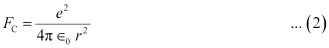Gravitational force of attraction between an electron and a proton is given as:

Where,

G = Gravitational constant = 6.67 × 10−11 N m2/kg2

If the electrostatic (Coulomb) force and the gravitational force between an electron and a proton are equal, then we can write:

FG = FC

Putting the value of equation (4) in equation (1), we get:

It is known that the universe is 156 billion light years wide or 1.5 × 1027 m wide. Hence, we can conclude that the radius of the first Bohr orbit is much greater than the estimated size of the whole universe.

Question 12.13:

Obtain an expression for the frequency of radiation emitted when a hydrogen atom de-excites from level to level (n−1). For large n, show that this frequency equals the classical frequency of revolution of the electron in the orbit.

It is given that a hydrogen atom de-excites from an upper level (n) to a lower level (n−1).

We have the relation for energy (E1) of radiation at level as:

Now, the relation for energy (E2) of radiation at level (− 1) is givenas: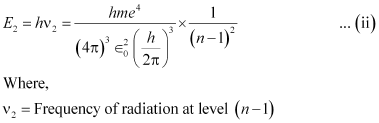Energy (E) released as a result of de-excitation:

E = E2E1

= E2 − E1 … (iii)

Where,

ν = Frequency of radiation emitted

Putting values from equations (i) and (ii) in equation (iii), we get:

For large n, we can write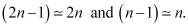Classical relation of frequency of revolution of an electron is given as: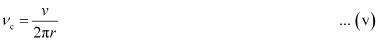Where,

Velocity of the electron in the nth orbit is given as:

v =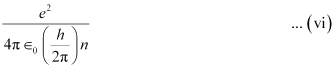And, radius of the nth orbit is given as:

r =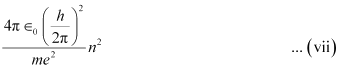Putting the values of equations (vi) and (vii) in equation (v), we get:

Hence, the frequency of radiation emitted by the hydrogen atom is equal to its classical orbital frequency.

Page No 437:

Question 12.14:

Classically, an electron can be in any orbit around the nucleus of an atom. Then what determines the typical atomic size? Why is an atom not, say, thousand times bigger than its typical size? The question had greatly puzzled Bohr before he arrived at his famous model of the atom that you have learnt in the text. To simulate what he might well have done before his discovery, let us play as follows with the basic constants of nature and see if we can get a quantity with the dimensions of length that is roughly equal to the known size of an atom (~ 10−10 m).

(a) Construct a quantity with the dimensions of length from the fundamental constants eme, and c. Determine its numerical value.

(b) You will find that the length obtained in (a) is many orders of magnitude smaller than the atomic dimensions. Further, it involves c. But energies of atoms are mostly in non-relativistic domain where is not expected to play any role. This is what may have suggested Bohr to discard and look for ‘something else’ to get the right atomic size. Now, the Planck’s constant h had already made its appearance elsewhere. Bohr’s great insight lay in recognising that hme, and will yield the right atomic size. Construct a quantity with the dimension of length from hme, and and confirm that its numerical value has indeed the correct order of magnitude.

(a) Charge on an electron, e = 1.6 × 10−19 C

Mass of an electron, me = 9.1 × 10−31 kg

Speed of light, c = 3 ×108 m/s

Let us take a quantity involving the given quantities as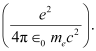Where,

0 = Permittivity of free space

And,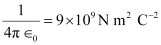The numerical value of the taken quantity will be:

Hence, the numerical value of the taken quantity is much smaller than the typical size of an atom.

(b) Charge on an electron, e = 1.6 × 10−19 C

Mass of an electron, me = 9.1 × 10−31 kg

Planck’s constant, h = 6.63 ×10−34 Js

Let us take a quantity involving the given quantities as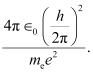Where,

0 = Permittivity of free space

And,The numerical value of the taken quantity will be:

Hence, the value of the quantity taken is of the order of the atomic size.

Question 12.15:

The total energy of an electron in the first excited state of the hydrogen atom is about −3.4 eV.

(a) What is the kinetic energy of the electron in this state?

(b) What is the potential energy of the electron in this state?

(c) Which of the answers above would change if the choice of the zero of potential energy is changed?

(a) Total energy of the electron, E = −3.4 eV

Kinetic energy of the electron is equal to the negative of the total energy.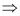K = −E

= − (− 3.4) = +3.4 eV

Hence, the kinetic energy of the electron in the given state is +3.4 eV.

(b) Potential energy (U) of the electron is equal to the negative of twice of its kinetic energy.U = −2 K

= − 2 × 3.4 = − 6.8 eV

Hence, the potential energy of the electron in the given state is − 6.8 eV.

(c) The potential energy of a system depends on the reference point taken. Here, the potential energy of the reference point is taken as zero. If the reference point is changed, then the value of the potential energy of the system also changes. Since total energy is the sum of kinetic and potential energies, total energy of the system will also change.

Question 12.16:

If Bohr’s quantisation postulate (angular momentum = nh/2π) is a basic law of nature, it should be equally valid for the case of planetary motion also. Why then do we never speak of quantisation of orbits of planets around the sun?

We never speak of quantization of orbits of planets around the Sun because the angular momentum associated with planetary motion is largely relative to the value of Planck’s constant (h). The angular momentum of the Earth in its orbit is of the order of 1070h. This leads to a very high value of quantum levels of the order of 1070. For large values of n, successive energies and angular momenta are relatively very small. Hence, the quantum levels for planetary motion are considered continuous.

Question 12.17:

Obtain the first Bohr’s radius and the ground state energy of a muonic hydrogen atom [i.e., an atom in which a negatively charged muon (μ) of mass about 207me orbits around a proton].

Mass of a negatively charged muon,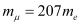According to Bohr’s model,

Bohr radius,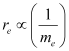And, energy of a ground state electronic hydrogen atom,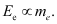We have the value of the first Bohr orbit,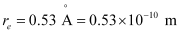Let  be the radius of muonic hydrogen atom.

At equilibrium, we can write the relation as:

Hence, the value of the first Bohr radius of a muonic hydrogen atom is

2.56 × 10−13 m.

We have,

Ee= − 13.6 eV

Take the ratio of these energies as:

Hence, the ground state energy of a muonic hydrogen atom is −2.81 keV.

## Conclusion

NCERT Solutions for Class 12 Physics Chapter 12 – Atoms, is a crucial chapter in the Class 12 Physics syllabus. It provides an in-depth understanding of the fundamental particles that make up an atom, the atomic models proposed by different scientists, and the structure of the atom. Through this chapter, students will learn about the Bohr’s model, the de Broglie hypothesis, the Heisenberg uncertainty principle, and the quantum mechanical model of the atom. The NCERT Solutions for Class 12 Physics Chapter 12 provide detailed explanations, which will help students to grasp the concepts effectively. The solutions also contain solved examples and practice questions that will help students to test their understanding of the chapter. By studying this chapter thoroughly, students can develop a strong foundation in atomic structure, which will be useful for further studies in physics and other related fields.

### Why do students prefer SWC for NCERT Solutions?

At SWC, students directly learn from IITians and experienced faculties. We provide quality study materials to prepare and follow a LEAP model. We teach not only for exams but for lifelong applications## FAQs Physics Class 12 Chapter 12

### What is an atom according to NCERT?

Atoms are the fundamental building units of all matter.

### What are atoms made of Class 12?

Protons, neutrons, and electrons.

### What are free electrons?

Electrons that are not permanently attached to an atom and take part in conduction are referred to as free electrons.

### How is an atom structure?

Atom is made up of a central nucleus with protons and neutrons; electrons orbit around the nucleus in definite energy shells.

Yes

## Summary

1. Both Thomson’s and Rutherford’s models represent an unstable system. Electrostatically, Thomson’s model is unstable, whereas Rutherford’s model is unstable due to electromagnetic radiation from orbiting electrons.
1. Bohr’s model of the hydrogen atom had an orbital image that contradicted the uncertainty principle. Modern quantum mechanics supplanted it, and Bohr’s orbits are places where the electron can be discovered with a high probability.
1. Unlike in the solar system, where planet-planet gravitational forces are negligible compared to the gravitational force of the sun on each planet (due to the sun’s mass being so much greater than the mass of any of the planets), the electron-electron electric force interaction is comparable in magnitude to the electronnucleus electrical force due to the same order of magnitude of charges and distances. This is why the Bohr model, which includes a planet-like electron, does not apply to many-electron atoms.
1. By postulating particular orbits in which electrons do not radiate, Bohr lay the groundwork for quantum theory. Only one quantum number, n, is included in Bohr’s model. Bohr’s postulate is supported by a new theory known as quantum mechanics. In quantum mechanics (the more widely recognized theory), however, a particular energy level may not correspond to a single quantum state. A state is defined by four quantum numbers (n, l, m, and s), although the energy of a pure Coulomb potential (as in the hydrogen atom) is solely determined by n.